The force acting on a body moving along x-axis varies with the position of the particle as shown in the fig.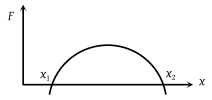The body is in stable equilibrium at

(1) x = x1

(2) x = x2

(3) both x1 and x2

(4) neither x1 nor x2

Concept Questions :-

Potential energy: Relation with force
High Yielding Test Series + Question Bank - NEET 2020

Difficulty Level:

The potential energy of a particle varies with distance x as shown in the graph. The force acting on the particle is zero at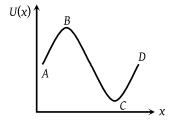(1) C

(2) B

(3) B and C

(4) A and D

Concept Questions :-

Potential energy: Relation with force
High Yielding Test Series + Question Bank - NEET 2020

Difficulty Level:

Figure shows the F-x graph. Where F is the force applied and x is the distance covered by the body along a straight line path. Given that F is in newton and x in metre, what is the work done ?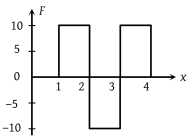(1) 10 J

(2) 20 J

(3) 30 J

(4) 40 J

Concept Questions :-

Work done by variable force
High Yielding Test Series + Question Bank - NEET 2020

Difficulty Level:

The force required to stretch a spring varies with the distance as shown in the figure. If the experiment is performed with the above spring of half length, the line OA will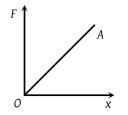(1) Shift towards F-axis

(2) Shift towards X-axis

(3) Remain as it is

(4) Become double in length

Concept Questions :-

Elastic potential energy
High Yielding Test Series + Question Bank - NEET 2020

Difficulty Level:

The graph between E and v is

(1)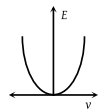(2)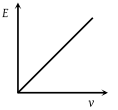(3)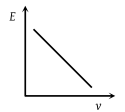(4)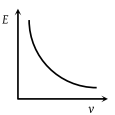Concept Questions :-

Work-Energy theorem
High Yielding Test Series + Question Bank - NEET 2020

Difficulty Level:

A body moves from rest with a constant acceleration. Which one of the following graphs represents the variation of its kinetic energy K with the distance travelled x ?

(1)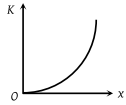(2)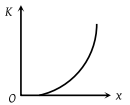(3)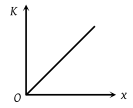(4)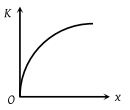High Yielding Test Series + Question Bank - NEET 2020

Difficulty Level:

The diagrams represent the potential energy U of a function of the inter-atomic distance r. Which diagram corresponds to stable molecules found in nature.

(1)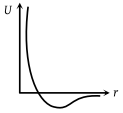(2)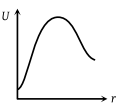(3)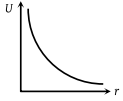(4)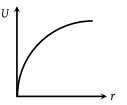Concept Questions :-

Potential energy: Relation with force
High Yielding Test Series + Question Bank - NEET 2020

Difficulty Level:

The relationship between the force F and position x of a body is as shown in figure. The work done in displacing the body from x = 1 m to x = 5 m will be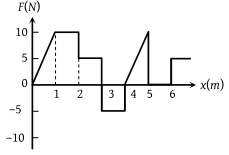(1) 30 J

(2) 15 J

(3) 25 J

(4) 20 J

Concept Questions :-

Work done by variable force
High Yielding Test Series + Question Bank - NEET 2020

Difficulty Level:

A particle is placed at the origin and a force F = kx is acting on it (where k is positive constant). If U(0) = 0, the graph of U(x) versus x will be (where U is the potential energy function)

(1)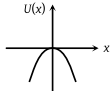(2)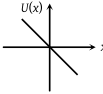(3)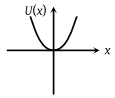(4)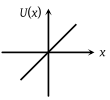Concept Questions :-

Elastic potential energy
High Yielding Test Series + Question Bank - NEET 2020

Difficulty Level:

A particle moves from a point $\left(-2\stackrel{^}{i}+5\stackrel{^}{j}\right)$ to $\left(4\stackrel{^}{j}+3\stackrel{^}{k}\right)$ when a force of  $\left(4\stackrel{^}{i}+3\stackrel{^}{j}\right)$ N is applied. How much work has been done by the force?

1. 8 J

2. 11 J

3. 5 J

4. 2 J

Concept Questions :-

Concept of work